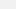The Kissing Circles: When 'Nature' Published Poetry

An ancient proof of a seemingly simple idea.

|
Feb 8 2015, 12:00pm​Image: Wiki

​Take a curve. Not quite any curve, but one we can call "sufficiently smooth." It has no sudden bends or joints, just a graceful arc in two dimensions. It could be anything.

There are lots of ways to describe this curve, which is what calculus is, basically: the study of change. But a curve is also a matter of space, and one way of describing a curve is with a circle. A curve is just a piece of a circle (some circle), after all.

The specific circle we want is a tangent circle, which is a geometric object that might be placed against a curve as below. It's a circle whose radius is determined by the momentary, infinitesimal curvature local to the point P. It can be nested on the "inside" of the curve or the outside, and sometimes both, if the circle is meant to trace a wave.Image: ​plus.maths.org

The key thing here is the "radius of curvature," which is the radius of a circular arc best describing a curve at a point (P). In this way, every part of every curve has a corresponding circle. A very shallow curve will have a very large corresponding circle (less curvature, larger radius), while a deep, extreme curve will conceal just the smallest circle. Imagine, for example, the curvature of the Earth beneath our feet, or the curvature of the whole of space-time—which is just the universe itself (being kissed).

This circle is also known as ​an osculating circle, from the Latin circulus osculans, which itself indeed translates to something like "kissing circle." Kissing circles, as we look around and consider our surrounding shapes and dimensions, start to look like much more than a geometrical novelty, and a necessary feature of space. Where there's a curve, there exists the completion of that curve, which is just another kissing circle. And everything is a curve, even a straight line, which is a curve without curvature.

The idea comes first from the ​Descartes theorem, which is a related statement about curvature describing how the radii of three touching circles (kissing, again) when plugged into a certain quadratic equation will result in a fourth circle, which "kisses" (is tangent to) all three, either from the inside-out or outside-in.

Descartes theorem was rediscovered in the early-1900s by Frederick Soddy, a chemist who won a Nobel Prize in 1921 for his work in radioactivity. In 1936, ​Soddy published "The Kiss Precise" in the scientific journal Nature, which begins like this:

For pairs of lips to kiss maybe
Involves no trigonometry.
This not so when four circles kiss
Each one the other three.
To bring this off the four must be
As three in one or one in three.
If one in three, beyond a doubt
Each gets three kisses from without.
If three in one, then is that one
Thrice kissed internally.

The idea behind Descartes theorem is that given three touching circles, we can produce a fourth one in either of these two configurations.Image: ​AMS

And we can keep going like this, adding more circles in a process called Apollonian packing, named after another of the great Greek geometry thinkers. And you can do this indefinitely, with trios of kissing circles producing new kissing circles. Forever.Which is a lot of kissing.